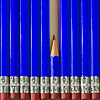# Short problems for Starters, Homework and Assessment

The links below take you to a selection of short problems based on UKMT junior and intermediate mathematical challenge questions. We have chosen these problems because they are ideal for consolidating and assessing subject knowledge, mathematical thinking and problem-solving skills. You may wish to use these as lesson starters, homework tasks, or as part of internal assessment exercises.

Longer NRICH problems can be found on the Secondary Curriculum page.### Number - Short Problems

A collection of short Stage 3 and 4 problems on number.### Algebra - Short Problems

A collection of short Stage 3 and 4 problems on algebra.### Geometry and Measure - Short Problems

A collection of short Stage 3 and 4 problems on geometry and measure.### Handling Data - Short Problems

A collection of short Stage 3 and 4 problems on handling data.### Working Mathematically - Short Problems

A collection of short Stage 3 and 4 problems on Working Mathematically.